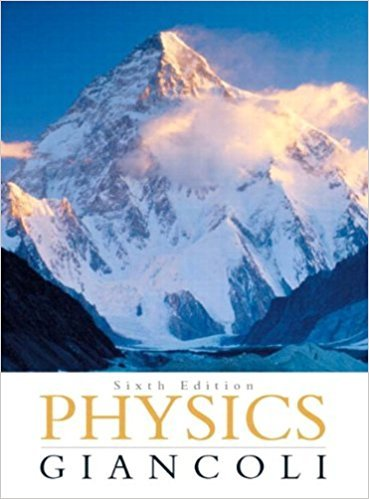×
×

# Solved: A hypothetical planet has a mass 1.66 times thatISBN: 9780321569837 99

## Solution for problem 32P Chapter 5

Physics: Principles with Applications | 6th Edition

• Textbook Solutions
• 2901 Step-by-step solutions solved by professors and subject experts
• Get 24/7 help from StudySoup virtual teaching assistantsPhysics: Principles with Applications | 6th Edition

4 5 1 382 Reviews
12
0
Problem 32P

A hypothetical planet has a mass 1.66 times that of Earth, but the same radius. What is g near its surface?

Step-by-Step Solution:

Solution 32P

Step 1 of 4</p>

Here we have to calculate the acceleration due to gravity on the surface of a hypothetical planet which has a mass 1.66 times the Earth’s mass, but having same radius as Earth.

Letandare the mass and radius of the Earth respectively. So the radius of the hypothetical planet will beand the mass of the planet will beStep 2 of 4</p>

The acceleration due to gravity on the surface of any planet is given by,……...1

Whereis the acceleration due to gravity on the surface of any planet,is universal gravitational constant,andare the mass and radius of the planet respectively.

Step 3 of 4

Step 4 of 4

##### ISBN: 9780321569837

Unlock Textbook Solution